# How to compute the net present value of an investment in ExcelIn this article, Maite CARNICERO MARTINEZ (ESSEC Business School, Global Bachelor of Business Administration, 2021-2022, exchange student from the University of Salamanca) explains how to compute the net present value of an investment in Excel.

When the time comes that one must choose what project to embark on, there are several measures to compare the available options, such as the internal rate of return, the payback method and the net present value (also known as the “discounted cash flow” method or DCF). In this article, I will focus on the last one of these tools, which is the preferred by most financial analysts.

A project is a temporary, unique and progressive endeavor to produce a tangible or intangible result, for instance, a new product or a competitive advantage. It normally entails the execution of some tasks over a period of time, conditioned to limitations related to cost, quality or performance. During its implementation, an initial investment and a series of cash flows are to be generated at different times. Some examples of projects are: developing a new service, building a factory, and implementing a new process.

The Net Present Value (NPV) compares the present value of the future cash flows with the investment made at the beginning. The computation of the present value uses a the required rate of return. It takes into account the time value of money, translating future cash flows into today’s value, since the buying power of money today is greater that the buying power of the same amount in the future.

The NPV is the basis of the discounted cash flow model (DCF) which allows investors to compare the initial cash flow of expenditure against the present value of future cash flows. It could be used to evaluate whether an important investment is worthwhile, but also in mergers and acquisitions and to compare companies, like Warren Buffet does, because once we have calculated the different NPVs we will know which investment has the biggest gain.

To sum up, the NPV allows us to do evaluate investments from a financial point of view and select the best one.

## Modelling of an investment

How can we calculate it?

The mathematical formula for the NPV is given by: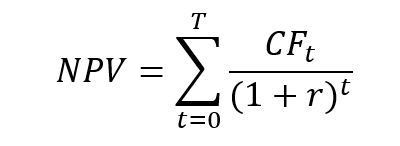CFt = cash flows of each period (from t=0 to t=T)
T = number of periods
r = discount rate or interested rate required of the investment. It is the rate of return that the investors expect on their investment

For a classical project, the first cash flow, CF0, is negative and corresponds to the initial cost of the project and the following cash flows, CFt for t=0 to t=T, are assumed to be positive. The NPV can be rewritten as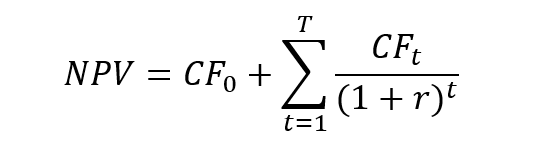This formula clearly shows that the NPV compares the first cash flow on the one hand, and the present value of future cash flows on the other hand.

## Investment decision

The NPV can be used as a criterion for the investment decision.

• If the NPV is positive, the investment should be made as it creates value.
• If the NPV is zero, the investment should be made or not.
• If the NPV is negative, the investment should not be made as it destroys value.

• The NPV of an investment is easy to calculate, specially nowadays with financial calculators and spreadsheets like Excel.
• The NPV measures the effect of the investment on the firm’s value.
• The NPV It takes into account the maturity of each cash flow.

• In order to compute the NPV, the discount rate has to be specified and it is a difficult issue.
• The calculations are based on assumptions and estimations and the reality can differ from them.
• Misestimations can be found in the initial investment, on the discount rate and on the projected returns of the project.
• The NPV formula presumes that the cash flows are immediately reinvested at the same rate as the discount rate.
• It presumes that the negative cash flows are financed with resources whose cost is also the discount rate.

## How to compute the NPV on Excel?

### Example

Excel is an extended tool in the financial world, also to calculate the NPV. Let’s take an example to illustrate how we can use it: we are offered a project in which we have to invest 42,000 euros and we will receive 8,400 euros the first year, 9,000 the second, 10,300 the third, 11,700 the fourth and 13,000 the last year.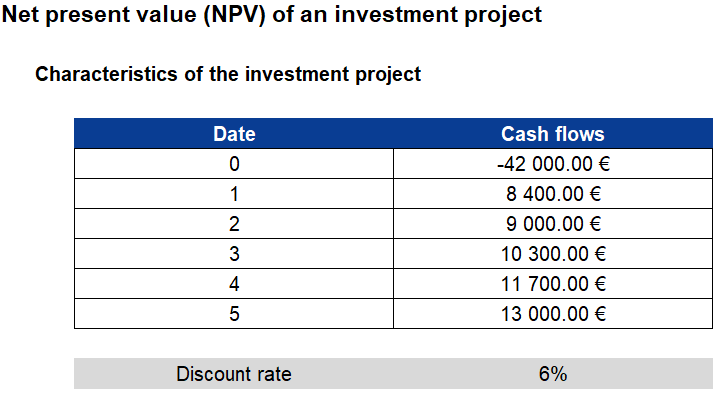Assuming that the discount rate is 6% per year, what will be the NPV?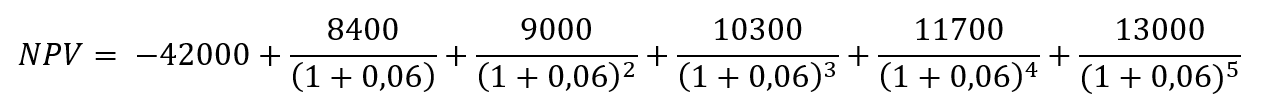We can do a hand-made computation of the NPV: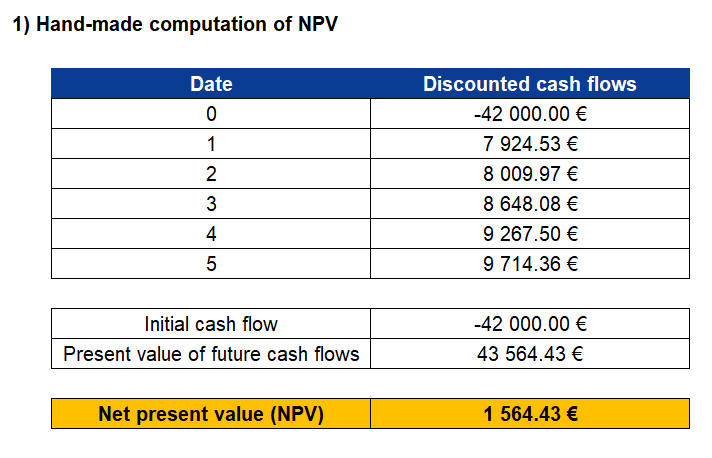We find a NPV of €1,564.43. As the NPV of the investment is positive, we will take the project.

### Computation with Excel

We can also use Excel to compute the NPV: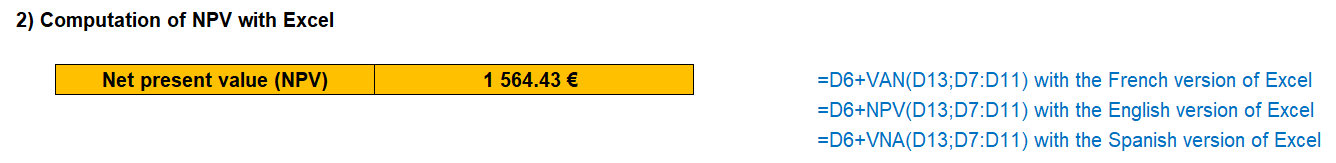## Useful resources

Mazars What is NPV?

Mazars NPV definition

Mazars NPV use and calculation

Mazars NPV limitations

Mazars Project definition

## Related posts on the SimTrade blog

▶ Chollat-Namy R. Bond valuation

▶ Fouques L. The IRR, XIRR and MIRR functions in Excel

▶ Paulen J. The IRR function in Excel

▶ Roero de Cortanze R. The Internal Rate of Return